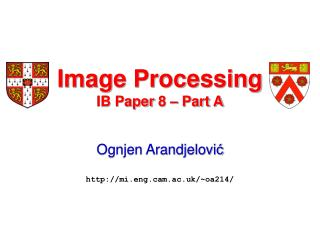DownloadDownload PresentationImage Processing IB Paper 8 – Part A

# Image Processing IB Paper 8 – Part A

Download Presentation## Image Processing IB Paper 8 – Part A

- - - - - - - - - - - - - - - - - - - - - - - - - - - E N D - - - - - - - - - - - - - - - - - - - - - - - - - - -
##### Presentation Transcript

1. Image ProcessingIB Paper 8 – Part A Ognjen Arandjelovićhttp://mi.eng.cam.ac.uk/~oa214/

2. Lecture Roadmap Face geometry • Lecture 1: Geometric image transformations  • Lecture 2: Colour and brightness enhancement  • Lecture 3: Denoising and image filtering  • Lecture 4: Cross-section through out-of-syllabustechniques

3. Filter Design – Matched Filters Consider the convolution sum of a discrete signal with a particular filter: 228+ 480+482+ 241 + … When is the filter response maximal?

4. Filter Design – Matched Filters The summation is the same as for vector dot product: The response is thus maximal when the two vectors are parallel i.e. when the filter matches the local patch it overlaps.

5. Filter Design – Intensity Discontinuities Using the observation that maximal filter response is exhibited when the filter matches the overlapping signal, we can start designing more complex filters: Kernel with maximal response to intensity edges 0.5 0.0 -0.5

6. Filter Design – Intensity Discontinuities Better yet, perform Gaussian smoothing to suppress noise first: Gaussian kernel Noise suppressing kernel with high response to intensity edges

7. + Unsharp Masking Enhancement The main principle of unsharp masking is to extract high frequency information and add it onto the original image to enhance edges: image output HPF Original edge Enhanced

8. Laplacian of Gaussian (LoG) Filter The Laplacian of Gaussian is an isotropic kernel that responds maximally to changes in the 2nd derivative: 1D Laplacian of Gaussian 2D LoG as a surface 2D LoG as an image 2D Laplacian of Gaussian:

9. Laplacian of Gaussian (LoG) Filter The response of the 1D Laplacian of Gaussian filter to an edge: Signal (edge) LoG filter Filter output -

10. Unsharp Masking Enhancement Unsharp mask filtering performs noise reduction and edge enhancement in one go, by combining a Gaussian LPF with a Laplacian of Gaussian kernel: + = Gaussian smoothing Convolution with –ve Laplacian of Gaussian Result

11. Unsharp Masking – Example Consider the following synthetic example: Gaussian smoothed then corrupted with Gaussian noise

12. Unsharp Masking – Example After unsharp masking: Gaussian smoothed then corrupted with Gaussian noise

13. – Distance Transform –

14. Motivation – Toy Problem The problem: produce output image with higher pixel value indicating higher level of belief that a roughly square polygon of edge 225 is centered at it: 225

15. Matched Filtering May Be? Given the material covered in the previous lecture, you may be tempted to create a matched filter: 225

16. Matched Filtering May Be? Here is the output of convolving the filter with the example image: A rather ugly looking result with too sharp discontinuities (i.e. low robustness to small deformations in shape, angle or thickness)

17. Distance Transform Rather, compute the distance transformed image – each pixel value indicates the minimal distance of that pixel to the nearest edge in the original image: Distance transformed Original image

18. Result after Distance Transform Consider now the result of convolving our matched filter with the distance transformed image: The result is far better looking!

19. – Nonlinear Denoising –

20. Salt and Pepper Noise Consider an image synthetically corrupted with salt and pepper noise: “Pepper”(dark) “Salt”(bright)

21. Salt and Pepper Noise Here is the result of denoising attempt using a Gaussian low-pass filter:

22. Median Filter Median filter replaces the old pixel value by the median of its neighbourhood: ± 3 pixel neighbourhood (sorted) 0 91 92 93 93 97 108 Original value Median

23. Median Filter – 1D Example The result of applying the median filter (with neighbourhood of size 7) on the corrupted 1D signal:

24. Median Filter – 2D Example The result of applying the median filter (mask size 3 х 3) on the synthetically corrupted image: Noise virtually entirely removed No edge smoothing

25. Filter Comparison The advantages of the median filter are easily seen when considering the difference to the ground truth: Median filtering Gaussian denoising RMS difference: 15 (from 20) RMS difference: 5 (from 20)

26. – De-Convolution –

27. An Example Problem Consider an image of a car plate acquired by a speed control camera: The plate is entirely unreadable due to motion blur Is it possible to somehow enhance this image to the level that the plate number can be read off?

28. Image Formation Model Assuming constant car velocity* the motion blur is caused by simple spatial averaging in the direction of apparent velocity. As before, this is equivalent to convolving the original, sharp image with a simple pulse function. * A reasonable assumption, given that the exposure is relatively short.

29. / Recovery Algorithm Given an estimate of the car velocity and our image formation model suggests the following algorithm: Degradation model 2D Fourier Transform De-blurred result 2D Fourier Transform

30. The Result Using the pulse width of 15 pixels produces the following de-blurred result: RE03 TGZ

31. – Super-Resolution –

32. What is Image Super-Resolution Given one or more low-resolution (LR) images, produce an enhanced, high-resolution (HR) image. • Observation model: Noise “True” image Observed LR image Transformation (geometric, photometric…)

33. SR via Non-Uniform Interpolation One of the simplest forms of super-resolution takes on the form of interpolation from non-aligned samples: Not quite the same

34. Example – 2x Sampling Frequency Simple scaling Non-uniform interpolation

35. – That is All for Today –